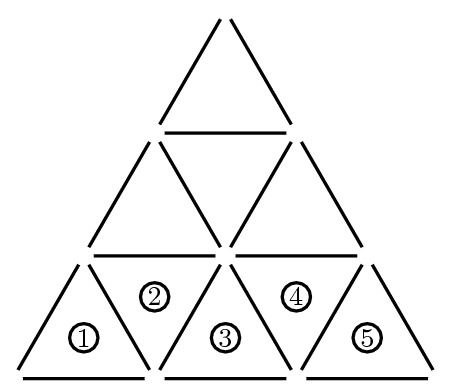###### back to index | new

Given that $a_n a_{n-2} - a_{n-1}^2 +a_n-na_{n-2}=-n^2+3n-1$ and $a_0=1$, $a_1=3$, find $a_{20}$.

On her first day of work, Janabel sold one widget. On day two, she sold three widgets. On day three, she sold five widgets, and on each succeeding day, she sold two more widgets than she had sold on the previous day. How many widgets in total had Janabel sold after working $20$ days?

Which of the following integers cannot be written as the sum of four consecutive odd integers?

An arithmetic sequence is a sequence in which each term after the first is obtained by adding a constant to the previous term. For example, $2,5,8,11,14$ is an arithmetic sequence with five terms, in which the first term is $2$ and the constant added is $3$. Each row and each column in this $5\times5$ array is an arithmetic sequence with five terms. What is the value of $X$?

Define the sequence $a_i$ as follows: $a_1 = 1, a_2 = 2015$, and $a_n =\frac{na_{n-1}^2}{a_{n-1}+na_{n-2}}$ for $n > 2$. What is the least $k$ such that $a_k < a_{k-1}$?

Suppose that $(u_n)$ is a sequence of real numbers satisfying $u_{n+2}=2u_{n+1}+u_n$, and that $u_3=9$ and $u_6=128$. What is $u_{2015}$?

Show that $1^{2017}+2^{2017}+\cdots + n^{2017}$ is not divisible by $(n+2)$ for any positive integer $n$.

Show that $$\sum_{k=0}^{n-1}(m+k)(m+k+1)=\frac{n}{3}(3m^2 +3mn+n^2-1)$$

Find the remainder when $1\times 2 + 2\times 3 + 3\times 4 + \cdots + 2018\times 2019$ is divided by $2020$.

Let sequence $\{a_n\}$ satisfy $a_0=0, a_1=1$, and $a_n = 2a_{n-1}+a_{n-2}$. Show that $2^k\mid n$ if and only if $2^k\mid a_n$.

Let $\{a_n\}$ be a sequence defined as $a_n=\lfloor{n\sqrt{2}}\rfloor$ where $\lfloor{x}\rfloor$ indicates the largest integer not exceeding $x$. Show that this sequence has infinitely many square numbers.

Let sequence $g(n)$ satisfy $g(1)=0, g(2)=1, g(n+2)=g(n+1)+g(n)+1$ where $n\ge 1$. Show that if $n$ is a prime greater than 5, then $n\mid g(n)[g(n)+1]$.

Show that all the terms of the sequence $a_n=\frac{(2+\sqrt{3})^n-(2-\sqrt{3})^n}{2\sqrt{3}}$ are integers, and also find all the $n$ such that $3 \mid a_n$.

Show that all terms of the sequence $a_n=\left(\frac{3+\sqrt{5}}{2}\right)^n+\left(\frac{3-\sqrt{5}}{2}\right)^n -2$ are integers. And when $n$ is even, $a_n$ can be expressed as $5m^2$, when $n$ is odd $a_n$ can be expressed as $m^2$.

If the $5^{th}$, $6^{th}$ and $7^{th}$ coefficients in the expansion of $(x^{-\frac{4}{3}}+x)^n$ form an arithmetic sequence, find the constant term in the expanded form.

Suppose that $a$ and $b$ are digits, not both nine and not both zero, and the repeating decimal $0.\overline{ab}$ is expressed as a fraction in lowest terms. How many different denominators are possible?

Consider the sequence of numbers: $4,7,1,8,9,7,6,\dots$ For $n>2$, the $n$-th term of the sequence is the units digit of the sum of the two previous terms. Let $S_n$ denote the sum of the first $n$ terms of this sequence. The smallest value of $n$ for which $S_n>10,000$ is:

Let $A_n$ be the average of all the integers between 1 and 101 which are the multiples of $n$ . Which is the largest among $A_2, A_3, A_4, A_5$ and $A_6$?

The sum of 2008 consecutive positive integers is a perfect square. What is the minimum value of the largest of these integers?

A large equilateral triangle is constructed by using toothpicks to create rows of small equilateral triangles. For example, in the figure we have $3$ rows of small congruent equilateral triangles, with $5$ small triangles in the base row. How many toothpicks would be needed to construct a large equilateral triangle if the base row of the triangle consists of $2003$ small equilateral triangles?Determine all polynomials such that $P(0) = 0$ and $P(x^2 + 1) = P(x)^2 + 1$.

A triangular array of $2016$ coins has $1$ coin in the first row, $2$ coins in the second row, $3$ coins in the third row, and so on up to $N$ coins in the $N$th row. What is the sum of the digits of $N$?

The sum of an infinite geometric series is a positive number $S$, and the second term in the series is $1$. What is the smallest possible value of $S?$

The sequence $(a_n)$ is defined recursively by $a_0=1$, $a_1=\sqrt{2}$, and $a_n=a_{n-1}a_{n-2}^2$ for $n\geq 2$. What is the smallest positive integer $k$ such that the product $a_1a_2\cdots a_k$ is an integer?

Let sequence $\{a_n\}$ satisfy the condition: $a_1=\frac{\pi}{6}$ and $a_{n+1}=\arctan(\sec a_n)$, where $n\in Z^+$. There exists a positive integer $m$ such that $\sin{a_1}\cdot\sin{a_2}\cdots\sin{a_m}=\frac{1}{100}$. Find $m$.